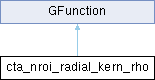GammaLib  1.7.0.dev

Kernel for zenith angle Nroi integration of radial model. More...

#include <GCTAResponse_helpers.hpp>## Public Member Functions

cta_nroi_radial_kern_rho (const GCTAResponseIrf *rsp, const GCTAObservation *obs, const GModelSpatialRadial *model, const GMatrix *rot, const GEnergy &srcEng, const GTime &srcTime, const GEnergy &obsEng, const GTime &obsTime, const double &dist, const double &radius, const double &omega0, const int &iter)

double eval (const double &rho)
Kernel for zenith angle Nroi integration or radial model. More...Public Member Functions inherited from GFunction
GFunction (void)
Void constructor. More...

GFunction (const GFunction &function)
Copy constructor. More...

virtual ~GFunction (void)
Destructor. More...

GFunctionoperator= (const GFunction &function)
Assignment operator. More...

## Protected Attributes

const GCTAResponseIrfm_rsp
CTA response. More...

const GCTAObservationm_obs
CTA observation. More...

const GMatrixm_rot
Rotation matrix. More...

GEnergy m_srcEng
True photon energy. More...

GTime m_srcTime
True photon arrival time. More...

GEnergy m_obsEng
Observed photon energy. More...

GTime m_obsTime
Observed photon arrival time. More...

double m_dist
Distance model-ROI centre. More...

double m_cos_dist
Cosine of distance model-ROI centre. More...

double m_sin_dist
Sine of distance model-ROI centre. More...

double m_omega0
Position angle of ROI. More...

int m_iter
Integration iterations. More...Protected Member Functions inherited from GFunction
void init_members (void)
Initialise class members. More...

void copy_members (const GFunction &function)
Copy class members. More...

void free_members (void)
Delete class members. More...

## Detailed Description

Kernel for zenith angle Nroi integration of radial model.

This class implements the integration kernel $$K(\rho)$$ for the integration

$\int_{\rho_{\rm min}}^{\rho_{\rm max}} K(\rho | E, t) d\rho$

of radial spatial models. The eval() method computes

$K(\rho | E, t) = \sin \rho \times S_{\rm p}(\rho | E, t) \times \int_{\omega_{\rm min}}^{\omega_{\rm max}} N_{\rm pred}(\rho,\omega) d\omega$

where

• $$S_{\rm p}(\rho | E, t)$$ is the radial model,
• $$N_{\rm pred}(\rho,\omega)$$ is the data space integral of the Instrument Response Function for a point spread function over the Region Of Interest,
• $$\rho$$ is the distance from the model centre, and
• $$\omega$$ is the azimuth angle is the position angle with respect to the connecting line between the model centre and the observed photon arrival direction.

Definition at line 322 of file GCTAResponse_helpers.hpp.

## Constructor & Destructor Documentation

 cta_nroi_radial_kern_rho::cta_nroi_radial_kern_rho ( const GCTAResponseIrf * rsp, const GCTAObservation * obs, const GModelSpatialRadial * model, const GMatrix * rot, const GEnergy & srcEng, const GTime & srcTime, const GEnergy & obsEng, const GTime & obsTime, const double & dist, const double & radius, const double & omega0, const int & iter )
inline

Definition at line 324 of file GCTAResponse_helpers.hpp.

## Member Function Documentation

 double cta_nroi_radial_kern_rho::eval ( const double & rho )
virtual

Kernel for zenith angle Nroi integration or radial model.

Parameters

Computes

$K(\rho | E, t) = \sin \rho \times S_{\rm p}(\rho | E, t) \times \int_{\omega_{\rm min}}^{\omega_{\rm max}} N_{\rm pred}(\rho,\omega) d\omega$

The azimuth angle integration range $$[\omega_{\rm min}, \omega_{\rm max}]$$ is limited to an arc around the vector connecting the model centre to the ROI centre. This limitation assures that the integration converges properly.

Implements GFunction.

Definition at line 534 of file GCTAResponse_helpers.cpp.

## Member Data Documentation

protected

Cosine of distance model-ROI centre.

Definition at line 362 of file GCTAResponse_helpers.hpp.

Referenced by eval().

protected

Definition at line 365 of file GCTAResponse_helpers.hpp.

Referenced by eval().

protected

Distance model-ROI centre.

Definition at line 361 of file GCTAResponse_helpers.hpp.

Referenced by eval().

protected

Integration iterations.

Definition at line 367 of file GCTAResponse_helpers.hpp.

Referenced by eval().

protected

Definition at line 355 of file GCTAResponse_helpers.hpp.

Referenced by eval().

protected

CTA observation.

Definition at line 354 of file GCTAResponse_helpers.hpp.

Referenced by eval().

protected

Observed photon energy.

Definition at line 359 of file GCTAResponse_helpers.hpp.

Referenced by eval().

protected

Observed photon arrival time.

Definition at line 360 of file GCTAResponse_helpers.hpp.

Referenced by eval().

protected

Position angle of ROI.

Definition at line 366 of file GCTAResponse_helpers.hpp.

Referenced by eval().

protected

Definition at line 364 of file GCTAResponse_helpers.hpp.

Referenced by eval().

protected

Rotation matrix.

Definition at line 356 of file GCTAResponse_helpers.hpp.

Referenced by eval().

protected

CTA response.

Definition at line 353 of file GCTAResponse_helpers.hpp.

Referenced by eval().

protected

Sine of distance model-ROI centre.

Definition at line 363 of file GCTAResponse_helpers.hpp.

Referenced by eval().

protected

True photon energy.

Definition at line 357 of file GCTAResponse_helpers.hpp.

Referenced by eval().